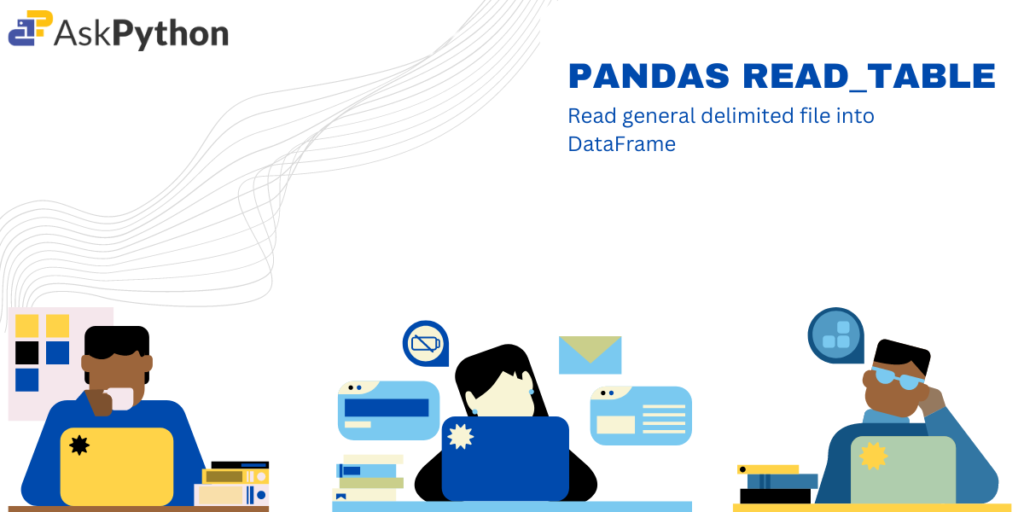In this article, we will learn about a pandas library ‘read_table()‘ which is used to read a file or string containing tabular data into a pandas DataFrame. The function is designed to handle many different file formats and data types, and it can be used to read data from a variety of sources, including text files, spreadsheets, databases, and more.

In the real world, we always come across different kinds of tabular data i.e a two-dimensional labeled data structure with columns and rows of potentially different types. Therefore for easy data analysis of such data, we need to convert it into pandas Dataframe. Hence, the `read_table()` function automatically performs many tasks, such as parsing and cleaning the data, converting values to the appropriate data type, and handling missing values, to make it easier to work with the resulting DataFrame.

## Syntax of pandas read_table() function

```pandas.read_table('string/path/file_name', delimiter=',')
```

There are a lot of parameters that can be used inside the read_table(). Some of the most used parameters are mentioned below.

We begin by importing the pandas library.

```import pandas as pd
```

### Example 1: Converting CSV file into Pandas Dataframe

```df= pd.read_table('/grades.csv',delimiter=',')
print(df)
```

We input a CSV file that stores the comma-separated values as data. We would convert the CSV file containing 9 columns to Pandas Dataframe.

### Example 2: Choosing which column to be used as row labels

```df = pd.read_table('/grades.csv', delimiter=',',index_col=1)
```

Using ‘index_col‘ parameter you can specify the index of the column(0-based indexing) you can specify which column should be used while parsing data and storing as a dataframe. For eg. column at index 1 which was ‘First name’ was selected as row label for all the entries.

### Example 3: Choosing which row to be used as column labels

```df = pd.read_table('/grades.csv', delimiter=',',header=3)
```

Using ‘header‘ parameter you can specify the index of the row(0-based indexing) you can specify which row should be used as a header while parsing data and storing as a dataframe. For eg. row at index 1 was selected header for all the entries.

### Example 4: Skipping rows from top keeping header

```df = pd.read_table('/grades.csv', delimiter=',',skiprows=range(1,4))

```

Using ‘skiprows‘ parameter you can specify the row numbers(0-based indexing) you can specify which rows should be skipped while parsing data and storing as a dataframe. For eg. rows from index 1 to 3 were skipped.

### Example 5: Skipping rows from the bottom of the table

```df = pd.read_table('/grades.csv', delimiter=',', skipfooter=5)
df.tail()
```

Using ‘skipfooter‘ parameter you can specify the number of rows from the bottom that should be skipped while parsing data and storing it as a dataframe. For eg. here last 5 rows were skipped(initially dataframe had 15 rows, but now there are only 5 rows).